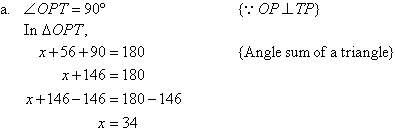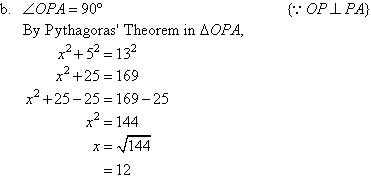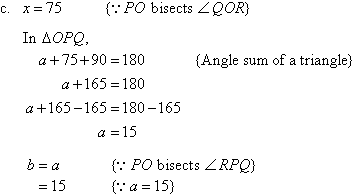Year 10 Interactive Maths - Second Edition

## Tangent to a Circle

A tangent to a circle is a straight line that touches a circle at one point only.

For example, PT is a tangent to the following circle at the point P. We call P the point of contact.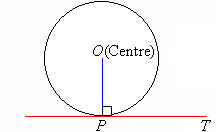###### Note:

A tangent is perpendicular to the radius at the point of contact.

#### Example 20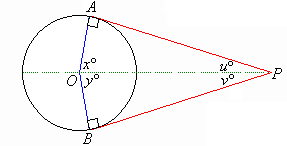If PA and PB are two tangents to a circle centred at O, prove that:

a.  PA = PB
b.  u = v
c.  x = y

##### Proof: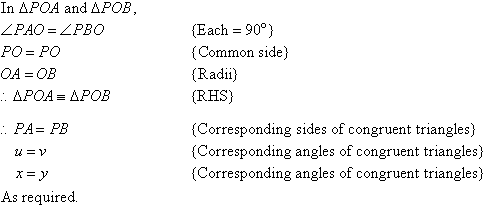###### In general:

If PA and PB are two tangents to a circle centred at O, then:

• the tangents to the circle from the external point P are equal
• OP bisects the angle between the two tangents
• OP bisects the angle between the two radii to the points of contact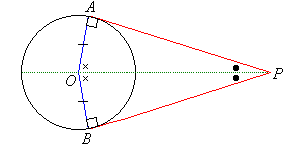#### Example 21

Find the value of the pronumeral(s) in each of the following diagrams:

a.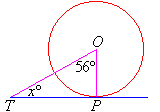b.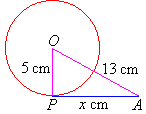c.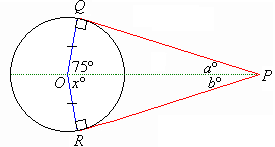##### Solution: